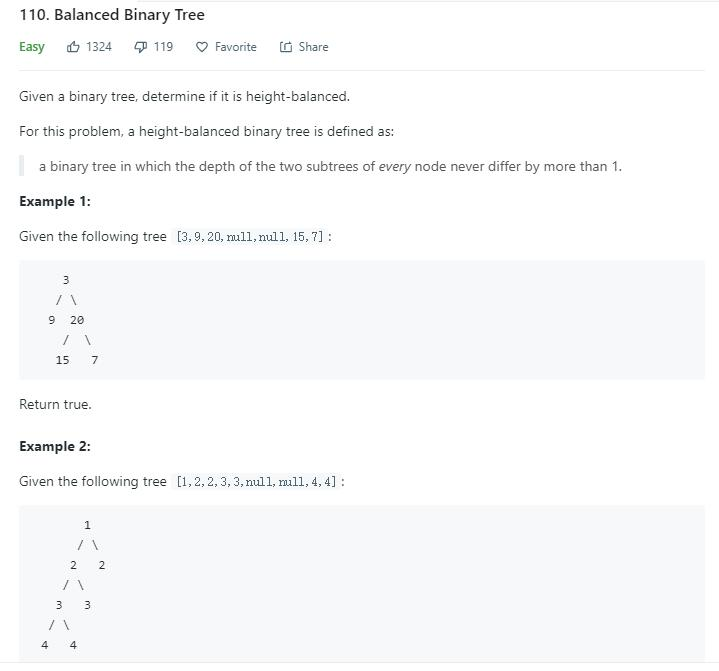# 题目描述（简单难度）# 解法一

public boolean isBalanced(TreeNode root) {
//它是一棵空树
if (root == null) {
return true;
}
//它的左右两个子树的高度差的绝对值不超过1
int leftDepth = getTreeDepth(root.left);
int rightDepth = getTreeDepth(root.right);
if (Math.abs(leftDepth - rightDepth) > 1) {
return false;
}
//左右两个子树都是一棵平衡二叉树
return isBalanced(root.left) && isBalanced(root.right);

}

private int getTreeDepth(TreeNode root) {
if (root == null) {
return 0;
}
int leftDepth = getTreeDepth(root.left);
int rightDepth = getTreeDepth(root.right);
return Math.max(leftDepth, rightDepth) + 1;
}


# 解法二

isBalanced(root.left) && isBalanced(root.right)


private int getTreeDepth(TreeNode root) {
if (root == null) {
return 0;
}
int leftDepth = getTreeDepth(root.left);
int rightDepth = getTreeDepth(root.right);
if (Math.abs(leftDepth - rightDepth) > 1) {
return -1;
}
return Math.max(leftDepth, rightDepth) + 1;
}


int leftDepth = getTreeDepth(root.left);
int rightDepth = getTreeDepth(root.right);


if (Math.abs(leftDepth - rightDepth) > 1) {
return -1;
}


private int getTreeDepth(TreeNode root) {
if (root == null) {
return 0;
}
int leftDepth = getTreeDepth(root.left);
if (leftDepth == -1) {
return -1;
}
int rightDepth = getTreeDepth(root.right);
if (rightDepth == -1) {
return -1;
}
if (Math.abs(leftDepth - rightDepth) > 1) {
return -1;
}
return Math.max(leftDepth, rightDepth) + 1;
}


public boolean isBalanced(TreeNode root) {
return getTreeDepth(root) != -1;
}

private int getTreeDepth(TreeNode root) {
if (root == null) {
return 0;
}
int leftDepth = getTreeDepth(root.left);
if (leftDepth == -1) {
return -1;
}
int rightDepth = getTreeDepth(root.right);
if (rightDepth == -1) {
return -1;
}
if (Math.abs(leftDepth - rightDepth) > 1) {
return -1;
}
return Math.max(leftDepth, rightDepth) + 1;
}